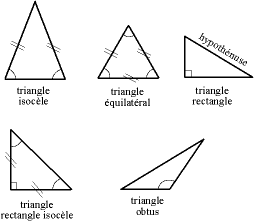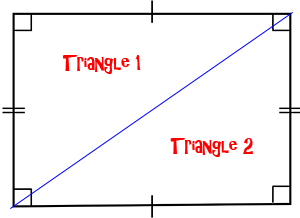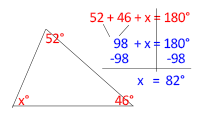# Triangle Sum Theorem Proof

Instructor: Danielle Wilson

Danielle is a certified elementary, middle school math, and special education teacher. She has a master's degree in elementary education and special education.

In this lesson, you'll learn about the triangle sum theorem proof, as well as the special trait all triangles share that can help you find missing angles in geometry problems.

## Triangle Sun Theorem

A triangle is a three-sided polygon with three angles. The sides of the triangle can be all the same length, or they can be all different lengths.

Equilateral triangles have all the same lengths and angles. Isosceles triangles have two lengths and angles that are the same and one length and angle that are different. A triangle with three different side lengths and angles is called a scalene triangle. A right triangle has one angle that is 90°.All triangles have one thing in common: all of their interior angles equal 180°. We call this common attribute the triangle sum theorem. Interior angles are the angles that you find inside of a polygon. If you add up the measure of each of the angles, you get the interior angle sum. The triangle sum theorem is very helpful in the study of geometry.

## Proving the Theorem

Any two triangles can be put together to make a quadrilateral. A quadrilateral is a four-sided polygon. A rectangle is a quadrilateral made up of four angles that each equal 90°. If we add up all of the angles of a rectangle, the total interior angle sum is 360°.If we cut this rectangle in half, moving from one vertex (or corner) to the next, it divides into two triangles. Since one of these triangles is half of the rectangle, the total interior angle sum of a triangle is half of 360°, or 180°. Mathematicians found this to be true of every type of quadrilateral, including trapezoids. Therefore all triangles must equal 180°.

## Find the Missing Angle

The triangle sum theorem is helpful for finding the missing angle in a triangle. When two angles are given, you can figure out the missing sum by doing the opposite operation. The sum of the angles will always equal 180°. Subtract the given angles from 180° to find the missing angle.

For example, the scalene triangle below has one angle that measures 46° and another angle that measures 52°. Subtract 46° and 52° from 180° to get the missing angle of 82°.To unlock this lesson you must be a Study.com Member.

### Register to view this lesson

Are you a student or a teacher?

#### See for yourself why 30 million people use Study.com

##### Become a Study.com member and start learning now.
Back
What teachers are saying about Study.com

### Earning College Credit

Did you know… We have over 200 college courses that prepare you to earn credit by exam that is accepted by over 1,500 colleges and universities. You can test out of the first two years of college and save thousands off your degree. Anyone can earn credit-by-exam regardless of age or education level.